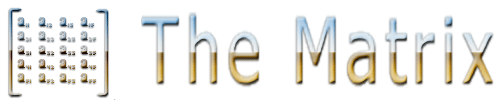#### Basic arithmetic operations on vectors and matrixes

Adding and subtracting vectors and matrixes is simply adding and subtracting appropriate elements. Multiplying vectors has two modifications: vector multiplication, when we have vector as a result, and scalar multiplication, when we have number as a result. Scalar multiplication is strongly connected with the conception of basis in multidimensional space and the result of it is projection of one vector onto another. It is given by simple formulae:

A*B = Ai*Bi

Note the simple rule: when one index is repeated several times in the expression, it means sum of all elements with this index

Vector multiplication is a bit more complicated. The result of it is the vector, ortogonal to both initial vectors, and the length of resulting vector is proportional to the square of triangle, created by two initial vectors. It is given by formulae:
[A,B]x = AyBz - AzBy
[A,B]y = AzBx - AxBz
[A,B]z = AxBy - AyBx
,or , using conception of determinants: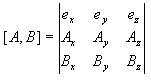Note that in 3-dimensional space vector multiplication involves 2 vectors, but in N-dimensional space it requires N-1 vectors of N dimensions, but scalar multiplication always requires only 2 vectors.

The most common form of linear dependence between vectors is:

Bi = AijBj

It is called multiplication of vector and matrix. Such multiplication performs translating vector from one basis to another, and elements of the matrix is scalar multiplications of every vector of old basis on every vector of new basis:

Aij = ei*ej

Matrix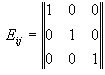is called Kroneker matrix, or single matrix. It's evident, that
AiEij = Aj

If we want to translate one vector first to one basis, and then to the second basis, we must multiplicate initial vector twice by the matrixes of this translations. The result may be considered as a result of multiplication on one single matrix, which is multiplication of two initial matrixes. So the result of multiplication of two matrixes is the matrix of the same dimension, and is given by formulae:

Cij = AikBljEkl
or C = A*B

The matrix Bij = Aji is called transponded matrix of A.

Here is the Delphi unit implementing basic operations on matrixes and vectors Matrix.pas.

#### Determinants, inverse matrixes and division by matrix.

In 3-dimensional space the square of triangle between two vectors is given by vector multiplication:
S = [A,B]
and the space of figure between three vectors is given by scalar multiplication of S on the third vector: V = C*S, or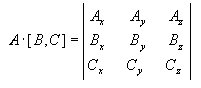In N-dimensional case the "N-dimensional space" of the figure between N vectors is evaluated as the determinant: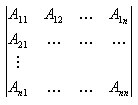A definition of determinant is recurrent: determinant of N-dimensional matrix is sum of mutiplications of i's element of the first string on determinant of initial matrix without first string and i's column. Remember to change sign on each next column.

Here is some properties of determinant:

• Determinant is not changed while transponing the matrix
• Determinant changes it's sign while swapping two rows or two columns of the matrix
• det(A*B) = det(A)*det(B)
• det(A-1) = det(A)-1
• When the basis given by matrix A is not full, det(A)=0

Let's try to find the matrix A-1 so that AA-1=1 The matrix A-1 is given by formulae:
Aij-1 = Sij /det(A) where Sij is an algebraic complementation of [i,j] element - the determinant of initial matrix without i's string and j's column and with sign, changed on each next column. Matrix A-1 is called inverse matrix. Multiplication AB-1 is called division A by B. Multiplication and division of matrixes is adding and subtracting of translations. For example, if you translated initial vector from one basis to another by multiplying it on matrix A, so you can translate it back by multiplying it on matrix A-1.

Here is the procedure evaluating determinant Determinant.pas and the Delphi unit implementing basic operations on matrixes and vectors Matrix.pas.

#### Applying matrix algebra in 3D-graphics

The most complicated areas of 3D-modelling is rotation of scenes and light emulation.

The rotations can be simply performed using polar system of coordinates. It's very simple to rotate objects in this coordinates, so rotating of the scene consists of several steps:

1. Getting new basis in polar coordinates. Tree basic vectors must be ortogonal to each other and the length of each vector must be 1
2. Translating each vector of this basis into decarte coordinates,using expressions:
x = R*cos(v)*cos(h)
y = R*cos(v)*sin(h)
z = R*sin(v)
where v and h is vertical angle of decline and horizontal angle of rotation.
3. Packing this three basic vectors into translation matrix.
4. Multiplying vectors of all points of the scene by this matrix
5. Redrawing scene
Rotation around one axis (Z) is given by such a translations:
Xn = X*cos(a) + Y*sin(a)
Yn = Y*cos(a) - X*sin(a)

Emulating light is based on the dependance between intensivities of initial and reflected rays, given by formulae:
I(a) = I0*cos(a), or, using vectors I = L*n,
where a is an angle of decline of element of the lighted surface, L is "light" vector
and n is the vector of single length, ortogonal to the surface.

Every 3D-object which can be lighted consists of many plate polygons (let's consider them triangles for simplification). To built the lighted scene, we must evaluate the color of each polygon. Let's assume that we have the coordinates of all points of triangles of our surface. Then emulating light can be performed in several steps:

1. Getting vectors of two sides of every triangle (see picture below)
2. Getting vector, normal to the surface of triangle by vector multiplying of this two vectors.
3. The length of this vector is being made 1 - so we get single vector normal to the surface (n).
4. By scalar multiplying n on the vector of light (it's direction is the direction of light ray and it's length is proportional to the intensivity of light) we get the intensivity of reflected light.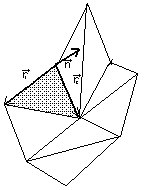RGB-components of color of our initial triangle is proportional to the intensivity of reflected light.

Here is the sample Delphi project demonstrating all this by building lighted three-dimensional surfaces Graph3D.zip

©2002-2003, Veter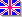English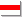Беларуская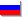Русский
Сайт создан в системе uCoz